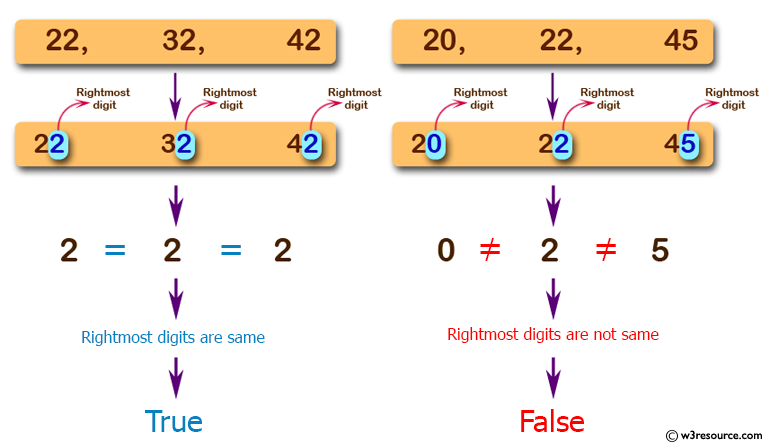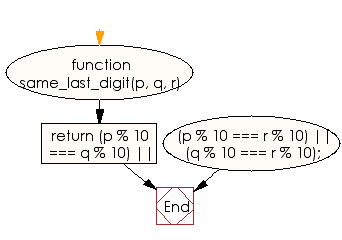# JavaScript: Check from three given numbers that two or all of them have the same rightmost digit

## JavaScript Basic: Exercise-43 with Solution

Write a JavaScript program to check from three given numbers (non negative integers) that two or all of them have the same rightmost digit.

Pictorial Presentation:Sample Solution:

HTML Code:

``````<!DOCTYPE html>
<html>
<meta charset="utf-8">
<meta name="viewport" content="width=device-width">
<title>JavaScript program to check from three  given numbers (non negative integers) that two  or all of them have the same rightmost digit.</title>
<body>
</body>
</html>
```
```

JavaScript Code:

``````function same_last_digit(p, q, r) {
return (p % 10 === q % 10) ||
(p % 10 === r % 10) ||
(q % 10 === r % 10);

}

console.log(same_last_digit(22,32,42));
console.log(same_last_digit(102,302,2));
console.log(same_last_digit(20,22,45));
```
```

Sample Output:

```true
true
false
```

Flowchart:ES6 Version:

``````function same_last_digit(p, q, r) {
return (p % 10 === q % 10) ||
(p % 10 === r % 10) ||
(q % 10 === r % 10);

}

console.log(same_last_digit(22,32,42));
console.log(same_last_digit(102,302,2));
console.log(same_last_digit(20,22,45));
``````

Live Demo:

See the Pen JavaScript: Check from three given numbers - basic-ex-43 by w3resource (@w3resource) on CodePen.

Improve this sample solution and post your code through Disqus

What is the difficulty level of this exercise?

Test your Programming skills with w3resource's quiz.

﻿

## JavaScript: Tips of the Day

Checks if a string is an anagram of another string (case-insensitive, ignores spaces, punctuation and special characters)

Example:

```const isAnagram = (str1, str2) => {
const normalize = str =>
str
.toLowerCase()
.replace(/[^a-z0-9]/gi, '')
.split('')
.sort()
.join('');
return normalize(str1) === normalize(str2);
};
console.log(isAnagram('iceman', 'cinema')); // true
```

Output:

```true
```